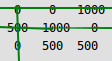# Hungarian Algorithm for Assignment Problem | Set 2 (Implementation)

• Difficulty Level : Expert
• Last Updated : 20 Jul, 2021

Given a 2D array, arr of size N*N where arr[i][j] denotes the cost to complete the jth job by the ith worker. Any worker can be assigned to perform any job. The task is to assign the jobs such that exactly one worker can perform exactly one job in such a way that the total cost of the assignment is minimized.

Example

Input: arr[][] = {{3, 5}, {10, 1}}
Output: 4
Explanation: The optimal assignment is to assign job 1 to the 1st worker, job 2 to the 2nd worker.
Hence, the optimal cost is 3 + 1 = 4.

Input: arr[][] = {{2500, 4000, 3500}, {4000, 6000, 3500}, {2000, 4000, 2500}}
Output: 4
Explanation: The optimal assignment is to assign job 2 to the 1st worker, job 3 to the 2nd worker and job 1 to the 3rd worker.
Hence, the optimal cost is 4000 + 3500 + 2000 = 9500.

Approach: The idea is to use the Hungarian Algorithm to solve this problem. The algorithm is as follows:

1. For each row of the matrix, find the smallest element and subtract it from every element in its row.
2. Repeat the step 1 for all columns.
3. Cover all zeros in the matrix using the minimum number of horizontal and vertical lines.
4. Test for Optimality: If the minimum number of covering lines is N, an optimal assignment is possible. Else if lines are lesser than N, an optimal assignment is not found and must proceed to step 5.
5. Determine the smallest entry not covered by any line. Subtract this entry from each uncovered row, and then add it to each covered column. Return to step 3.

Consider an example to understand the approach:

Let the 2D array be:

2500 4000 3500
4000 6000 3500
2000 4000 2500

Step 1: Subtract minimum of every row. 2500, 3500 and 2000 are subtracted from rows 1, 2 and 3 respectively.

0   1500  1000
500  2500   0
0   2000  500

Step 2: Subtract minimum of every column. 0, 1500 and 0 are subtracted from columns 1, 2 and 3 respectively.

0    0   1000
500  1000   0
0   500  500

Step 3: Cover all zeroes with minimum number of horizontal and vertical lines.Step 4: Since we need 3 lines to cover all zeroes, the optimal assignment is found.

2500  4000  3500
4000  6000  3500
2000  4000  2500

So the optimal cost is 4000 + 3500 + 2000 = 9500

For implementing the above algorithm, the idea is to use the max_cost_assignment() function defined in the dlib library. This function is an implementation of the Hungarian algorithm (also known as the Kuhn-Munkres algorithm) which runs in O(N3) time. It solves the optimal assignment problem.

Below is the implementation of the above approach:

## Python

 `# Python program for the above approach ` `import` `dlib ` ` `  `# Function to find out the best ` `# assignment of people to jobs so that ` `# total cost of the assignment is minimized ` `def` `minCost(arr): ` ` `  `    ``# Call the max_cost_assignment() function ` `    ``# and store the assignment ` `    ``assignment ``=` `dlib.max_cost_assignment(arr) ` ` `  `    ``# Print the optimal cost ` `    ``print``(dlib.assignment_cost(arr, assignment)) ` ` `  ` `  `# Driver Code ` ` `  `# Given 2D array ` `arr ``=` `dlib.matrix([[``3``, ``5``], [``10``, ``1``]]) ` ` `  `# Function Call ` `minCost(arr) `

Output

`4`

Time Complexity: O(N3)
Auxiliary Space: O(N2)

My Personal Notes arrow_drop_up
Recommended Articles
Page :